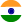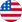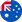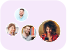24*7 support on WhatsAppChat NowProduct Edition:2nd Edition
Author: Julian J. Faraway
Book Name: Extending the Linear Model with R
Subject Name: Engineering

# Extending the Linear Model with R 2nd Edition Solutions

0 out of 5.0
53 reviews31 Students
have requested for homework help from this book

Start Analyzing a Wide Range of Problems Since the publication of the bestselling highly recommended first edition R has considerably expanded both in popularity and in the number of packages available. Extending the Linear Model with R: Generalized Linear Mixed Effects and Nonparametric Regression Models Second Edition takes advantage of the greater functionality now available in R and substantially revises and adds several topics. New to the Second Edition Expanded coverage of binary and binomial responses including proportion responses quasibinomial and beta regression and applied considerations regarding these models New sections on Poisson models with dispersion zero inflated count models linear discriminant analysis and sandwich and robust estimation for generalized linear models (GLMs) Revised chapters on random effects and repeated measures that reflect changes in the lme4 package and show how to perform hypothesis testing for the models using other methods New chapter on the Bayesian analysis of mixed effect models that illustrates the use of STAN and presents the approximation method of INLA Revised chapter on generalized linear mixed models to reflect the much richer choice of fitting software now available Updated coverage of splines and confidence bands in the chapter on nonparametric regression New material on random forests for regression and classification Revamped R code throughout particularly the many plots using the ggplot2 package Revised and expanded exercises with solutions now included Demonstrates the Interplay of Theory and Practice This textbook continues to cover a range of techniques that grow from the linear regression model. It presents three extensions to the linear framework: GLMs mixed effect models and nonparametric regression models. The book explains data analysis using real examples and includes all the R commands necessary to reproduce the analyses.Read more

5
27
4
11
3
15
2
12
1
0

0

## Students who viewed this book also checked out